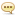Tutorials :

Technics Publications - Python Library Series The Definitive Guide to PyTorch-RiDWARE

Author: Minhchick   |   09 October 2019   |: 0Technics Publications - Python Library Series The Definitive Guide to PyTorch-RiDWARE
English | Size: 309.20 MB
Category: Tutorial

Dhiraj, a data scientist and machine learning evangelist, continues his teaching of Python libraries by explaining through both lecture and practice the PyTorch library.

Click here to watch all of Dhiraj Kumar's courses including the full Python Library Series.

In this course, become adept with the PyTorch library through these seven topics:

Introducing PyTorch. This first topic in the Python Library series introduces this fast and flexible deep learning framework produced by FaceBook. Learn all about this open source machine learning framework and its uses within computer vision and natural language processing. Apart from the Python interface, PyTorch also has a C++ frontend. Be able to explain tensors, variables, Autograd, and optimizers. Know the functionality of the torch.nn PyTorch module, which exposes neural-network specific functionality.
PyTorch Advantages. Know the advantages of PyTorch in this second topic in the Python Library series. PyTorch is used frequently for deep learning and artificial intelligence applications because it is Pythonic, easy to learn, well-documented, easy to debug, able to provide data parallelism, dynamic graph supportable, and able to export models in the Standard Open Neural Network Exchange Format (ONNX).
PyTorch Tensors. Create CPU and GPU tensors in this third topic in the Python Library series. A scalar is zero-dimensional, a vector is one-dimensional, a matrix is two-dimensional, and a tensor is three or more dimensions. PyTorch tensors are similar to numpy arrays with the additional features that tensors can be used on a GPU to accelerate computing.
PyTorch Autograd. Install and then apply automatic differentiation using autograd (automatic gradient) in this fourth topic in the Python Library series.
PyTorch vs. Tensorflow. Contrast PyTorch with TensorFlow in areas of functionality, performance, debugging, and visualization in this fifth topic in the Python Library series. PyTorch is a deep learning framework based on Torch. Tensorflow is an open source deep learning framework based on Theano. Tensorflow defines a computational graph statically before a model can run. PyTorch is much more dynamic with computational graphs than Tensorflow.
PyTorch vs. Keras. Contrast PyTorch with Keras in areas of functionality, performance, cross-platform, debugging, and visualization in this sixth topic in the Python Library series. Keras is a high-level application programming interface that sits on top of other deep learning frameworks such as Tensorflow.
PyTorch Linear Regression. Perform linear regression in this seventh topic in the Python Library series. Linear Regression is an algorithm that finds a linear relationship between a dependent variable and an independent variable. It is a statistical method that allows us to determine the relationship between two continuous variables.

R E L E A S E N O T E S, 1 - Unpack, 2 - Burn or Mount the ISO, 3 - Open the tutorial, 4 - Enjoy

G R E E T S

C O N T A C T U S

Buy Long-term Premium Accounts To Support Me & Max Speed